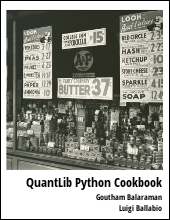Provides an introduction to valuation of convertible bonds using QuantLib Python with a minimal example.

Visit here for other QuantLib Python examples. If you found these posts useful, please take a minute by providing some feedback.

In :
import QuantLib as ql


In this blog I will work through an example of valuing convertible bonds in QuantLib. Lets start by doing the usual setup of creating a calculation_date and setting it as the evaluationDate.

In :
calculation_date = ql.Date(9,1,2004)
ql.Settings.instance().evaluationDate = calculation_date


One little quirk in the QuantLib convertible bond implementation is that there are places where the redemption amount is hard coded to 100. So if you have conversion ratio evaluated as \begin{eqnarray} Conversion\ Ratio = \frac{Redemption\ Amount}{Conversion\ Price} \end{eqnarray}

you will need to scale to an appropriate value with a redemption amount of 100. For instance, vendors report conversion ratio with a redemption amount of 1000. The conversion ratio obtained this way should be divided by a factor of 10 to get the equivalent conversion ratio for use in the QuantLib calculations. This is a limitation right now (as of version 1.7), which can be fixed in the future.

Following is the details of the convertible bond of interest.

In :
# St. Mary Land & Exploration Company
# Bloomberg ticker: SM 5.75 03/15/22

redemption = 100.00
face_amount = 100.0
spot_price = 29.04
conversion_price = 26.0
conversion_ratio = 3.84615  # BBG quotes 38.4615; had to scale by a factor of 10

issue_date = ql.Date(15,3,2002)
maturity_date = ql.Date(15,3,2022)

settlement_days = 2
calendar = ql.UnitedStates(ql.UnitedStates.GovernmentBond)
coupon = 0.0575
frequency = ql.Semiannual
tenor = ql.Period(frequency)

day_count = ql.Thirty360()

call_dates = [ql.Date(20,3,2007)]
call_price = 100.0
put_dates = [ql.Date(20,3,2007), ql.Date(15,3,2012), ql.Date(15,3,2017)]
put_price = 100.0

# assumptions
dividend_yield = 0.02
risk_free_rate = 0.04
volatility = 0.40


The call and put schedule for this bond is created as shown below. Here for each call date, we create a CallabilityPrice, then use that to form the Callability object. This is appended to the CallabilitySchedule object to form a list of call and put schedules.

In :
callability_schedule = ql.CallabilitySchedule()

for call_date in call_dates:
callability_price  = ql.CallabilityPrice(call_price,
ql.CallabilityPrice.Clean)
callability_schedule.append(ql.Callability(callability_price,
ql.Callability.Call,
call_date)
)

for put_date in put_dates:
puttability_price = ql.CallabilityPrice(put_price,
ql.CallabilityPrice.Clean)
callability_schedule.append(ql.Callability(puttability_price,
ql.Callability.Put,
put_date))


Any dividend information for the underlying stock is used to form the DividendSchedule.

In :
dividend_schedule = ql.DividendSchedule() # No dividends
dividend_amount = dividend_yield*spot_price
next_dividend_date = ql.Date(1,12,2004)
dividend_amount = spot_price*dividend_yield
for i in range(4):
dividend_schedule.append(
ql.FixedDividend(dividend_amount, date)
)


Now we bulid the fixed coupon convertible bond object

In :
schedule = ql.Schedule(issue_date, maturity_date, tenor,
calendar, accrual_convention, accrual_convention,
ql.DateGeneration.Backward, False)

exercise = ql.AmericanExercise(calculation_date, maturity_date)

convertible_bond = ql.ConvertibleFixedCouponBond(exercise,
conversion_ratio,
dividend_schedule,
callability_schedule,
issue_date,
settlement_days,
[coupon],
day_count,
schedule,
redemption)


Build the Black-Scholes-Merton process to model the equity part

In :
spot_price_handle = ql.QuoteHandle(ql.SimpleQuote(spot_price))
yield_ts_handle = ql.YieldTermStructureHandle(
ql.FlatForward(calculation_date, risk_free_rate, day_count)
)
dividend_ts_handle = ql.YieldTermStructureHandle(
ql.FlatForward(calculation_date, dividend_yield, day_count)
)
volatility_ts_handle = ql.BlackVolTermStructureHandle(
ql.BlackConstantVol(calculation_date, calendar,volatility, day_count)
)

bsm_process = ql.BlackScholesMertonProcess(spot_price_handle,
dividend_ts_handle,
yield_ts_handle,
volatility_ts_handle)


Build the convertible bond pricing engine

In :
time_steps = 1000
engine = ql.BinomialConvertibleEngine(bsm_process, "crr", time_steps)


Price the bond

In :
convertible_bond.setPricingEngine(engine)
print "NPV ", convertible_bond.NPV()

NPV  132.308276818